# Fraction Word Problems

#### Number of problems found: 617

• Tank 11When 150 litres has been drawn from a tank it is 3/8 full, how many litres will the tank hold?
• Three machines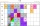The power of the three machines is 2: 3: 5. Two most powerful machines produce 400 parts per hour. How many components make all three machines in 3 hours?
• Marriage sttusIn our city, there are 3/5 of the women married to 2/3 of the men. Find what part of the population is free.
• Cups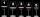We have three cups. In the cups we had fluid and boredom we started to shed. 1 We shed one-third of the fluid from the second glass into the first and third. 2 Then we shed one quarter cup of liquid from the first to the second and to the third. 3 Then we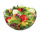We need two tenths kg of a carrot, one tenth of peas and three tenths of of tomatoes to make salad. Express the fraction of the weight of the vegetables to be salad. Convert the result to grams.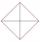In the square ABCD point P is in the middle of the DC side and point Q in the middle pages AD. If the area of quadrilateral BQPC is 49 cm2, what is the area of ABCD?
• To improper fractionChange mixed number to improper fraction a) 1 2/15 b) -2 15/17
• Points on line segmentPoints P & Q belong to segment AB. If AB=a, AP = 2PQ = 2QB, find the distance: between point A and the midpoint of the segment QB.
• Above EarthTo what height must a boy be raised above the earth in order to see one-fifth of its surface.
• Trip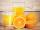On the trip drank 3/10 of pupils tea, 2/5 cola, 1/4 mineral water and remaining 3 juice. How many students were on the trip?
• PlayingHow long have we trained on the pitch when we know that the warm-up took 10 minutes, we trained passes for one-third of the time and we played football half the time?
• Pizza 4Marcus ate half pizza on monday night. He than ate one third of the remaining pizza on Tuesday. Which of the following expressions show how much pizza marcus ate in total?
• Car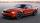Car goes from point A to point B at speed 86 km/h and back 53 km/h. If they goes there and back at speed 67 km/h trip would take 10 minutes shorter. What is distance between points A and B?
• 3 children3 children had to divide 4 pounds is candy. How much candy did each child get?
• The balls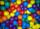You have 108 red and 180 green balls. You have to be grouped into the bags so that the ratio of red to green in each bag was the same. What smallest number of balls may be in one bag?
• Bridge piers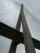One quarter of the bridge pier is sunk into the ground. Two thirds are in the water. Protruding above the water is 1.20 m long. Determine the height of bridge piers.The radius of the sphere we reduce by 1/3 of the original radius. How much percent does the volume and surface of the sphere change?
• Percentages 5\$5.25 is 7 1/2% of what number?
• Sixty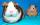Sixty percent of one-fifteen of the total is equal to thirty. What are two percent of the total?
• STRESSED wordEach letter in STRESSED is printed on identical cards, one letter per card and assembled in random order. Calculate the probability that the cards spell DESSERTS when assembled.

Do you have an interesting mathematical word problem that you can't solve it? Submit a math problem, and we can try to solve it.

We will send a solution to your e-mail address. Solved examples are also published here. Please enter the e-mail correctly and check whether you don't have a full mailbox.

Please do not submit problems from current active competitions such as Mathematical Olympiad, correspondence seminars etc...

Need help calculate sum, simplify or multiply fractions? Try our fraction calculator.